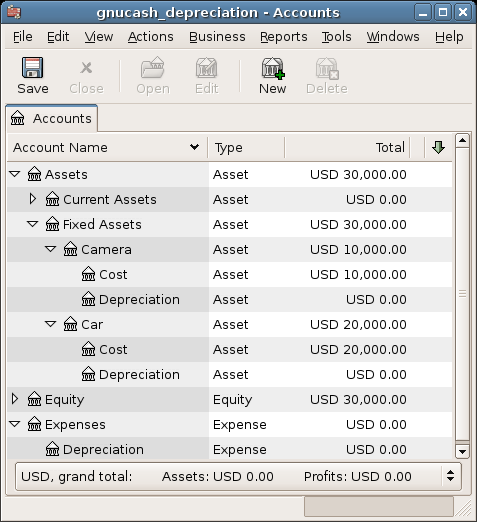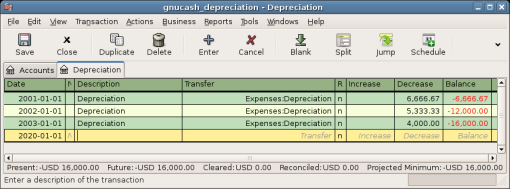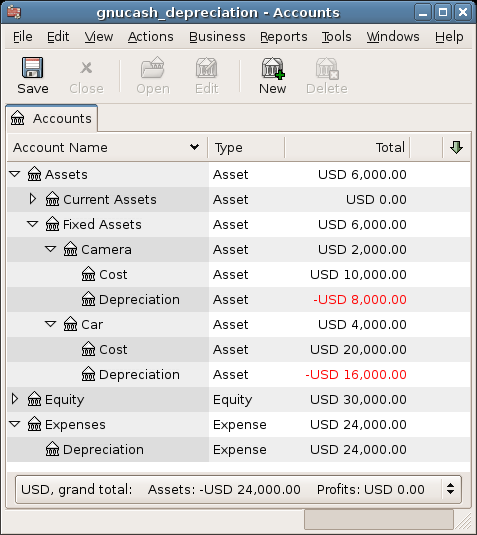# Depreciation¶

This chapter will introduce the concept of depreciation in accounting and give some real life examples for using it.

## Basic Concepts¶

Depreciation is the accounting method for expensing capital purchases over time. There are two reasons that you may want to record depreciation; you are doing bookkeeping for your own personal finances and would like to keep track of your net worth, or you are doing bookkeeping for a small busines and need to produce a financial statement from which you will prepare your tax return.

The method of recording depreciation is the same in either case. but the end goal is different. This section will discuss the differences between the two. But first, some terminology.

• Accumulated depreciation - the accumulated total of book depreciation taken over the life of the asset. This is accumulated in the depreciation account in the asset section.

• Book depreciation - this is the amount of depreciation that you record in your financial statements per accounting period.

• Fair market value - the amount for which an asset could be sold at a given time.

• Net book value - this is the difference between the original cost and the depreciation taken to date.

• Original cost - this is the amount that the asset cost you to purchase. It includes any cost to get the asset into a condition in which you can use it. For example - shipping, installation costs, special training.

• Salvage value - this is the value that you estimate the asset can be sold for at the end of it’s useful life (to you).

• Tax depreciation - this is the amount of depreciation that you take for income tax purposes.

### Personal Finances¶

Depreciation is used in personal finances to periodically lower an asset’s value to give you an accurate estimation of your current net worth. For example, if you owned a car you could keep track of its current value by recording depreciation every year. To accomplish this, you record the original purchase as an asset, and then record a depreciation expense each year (See Example for an example). This would result in the net book value being approximately equal to the fair market value of the asset at the end of the year.

Depreciation for personal finance has no tax implications, it is simply used to help you estimate your net worth. Because of this, there are no rules for how you estimate depreciation, use your best judgement.

For which assets should you estimate depreciation? Since the idea of depreciation for personal finances is to give you an estimate of your personal net worth, you need only track depreciation on assets of notable worth that you could potentially sell, such as a car or boat.

As opposed to personal finance where the goal is tracking personal worth, business is concerned with matching the expense of purchasing capital assets with the revenue generated by them. This is done through book depreciation. Businesses must also be concerned with local tax laws covering depreciation of assets. This is known as tax depreciation. The business is free to choose whatever scheme it wants to record book depreciation, but the scheme used for tax depreciation is fixed. More often than not this results in differences between book and tax depreciation, but steps can be taken to reduce these differences.

Now, what purchases should be capitalized? If you expect something that you purchase to help you earn income for more than just the current year, then it should be capitalized. This includes things like land, buildings, equipment, automobiles, and computers - as long as they are used for business purposes. It does not include items that would be considered inventory. So if you made a purchase with the intent to resell the item, it should not be capitalized.

In addition to the purchase of the asset itself, any costs associated with getting the asset into a condition so that you can use it should be capitalized. For example, if you buy a piece of equipment and it needs to be shipped from out of town, and then some electrical work needs to be done so you can plug the machine in, and some specialized training is needed so you know how to use the machine, all these costs would be included in the cost of the equipment.

You also need to know the estimated salvage value of the asset. Generally, this is assumed to be zero. The idea behind knowing the salvage value is that the asset will be depreciated until the net book value (cost less depreciation) equals the salvage value. Then, when the asset is written off, you will not have a gain or loss resulting from the disposal of the asset.

The last step is to determine the method of depreciation that you want to use. This will be discussed on the next few pages.

Warning

Be aware that different countries can have substantially different tax policies for depreciation; all that this document can really provide is some of the underlying ideas to help you apply your “favorite” tax/depreciation policies.

## Estimating Valuation¶

A central issue with depreciation is to determine how you will estimate the future value of the asset. Compared to the often uncertain estimates one has to do where appreciation of assets is concerned, we are on somewhat firmer ground here. Using sources listed below should make it fairly straight forward to estimate the future value of your depreciating assets.

• Tax Codes: For businesses that want to use depreciation for tax purposes, governments tend to set up precise rules as to how you are required to calculate depreciation. Consult your local tax codes, which should explicitly state how to estimate depreciation.

• Car Blue Book: For automobiles, it is easy to look up in references such as “Blue Books” estimates of what an automobile should be worth after some period of time in the future. From this you will be able to develop a model of the depreciation.

### Depreciation Schemes¶

A depreciation scheme is a mathematical model of how an asset will be expensed over time. For every asset which undergoes depreciation, you will need to decide on a depreciation scheme. An important point to keep in mind is that, for tax purposes, you will need to depreciate your assets at a certain rate. This is called tax depreciation. For financial statement purposes you are free to choose whatever method you want. This is book depreciation. Most small businesses use the same rate for tax and book depreciation. This way there is less of a difference between your net income on the financial statements and your taxable income.

This section will present 3 of the more popular depreciation schemes: linear, geometric, and sum of digits. To simplify the examples, we will assume the salvage value of the asset being depreciated is zero. If you choose to use a salvage value, you would stop depreciating the asset once the net book value equals the salvage value.

1. Linear depreciation diminishes the value of an asset by a fixed amount each period until the net value is zero. This is the simplest calculation, as you estimate a useful lifetime, and simply divide the cost equally across that lifetime.

Example: You have bought a computer for $1500 and wish to depreciate it over a period of 5 years. Each year the amount of depreciation is$300, leading to the following calculations:

 Year Depreciation Remaining Value 0 1500 1 300 1200 2 300 900 3 300 600 4 300 300 5 300 0
2. Geometric depreciation is depreciated by a fixed percentage of the asset value in the previous period. This is a front-weighted depreciation scheme, more depreciation being applied early in the period. In this scheme the value of an asset decreases exponentially leaving a value at the end that is larger than zero (i.e.: a resale value).

Example: We take the same example as above, with an annual depreciation of 30%.

 Year Depreciation Remaining Value 0 1500 1 450 1050 2 315 735 3 220.50 514.50 4 154.35 360.15 5 108.05 252.10

Note

Beware: Tax authorities may require (or allow) a larger percentage in the first period. On the other hand, in Canada, this is reversed, as they permit only a half share of “Capital Cost Allowance” in the first year. The result of this approach is that asset value decreases more rapidly at the beginning than at the end which is probably more realistic for most assets than a linear scheme. This is certainly true for automobiles.

3. Sum of digits is a front-weighted depreciation scheme similar to the geometric depreciation, except that the value of the asset reaches zero at the end of the period. This is a front-weighted depreciation scheme, more depreciation being applied early in the period. This method is most often employed in Anglo/Saxon countries. Here is an illustration:

Example: First you divide the asset value by the sum of the years of use, e.g. for our example from above with an asset worth $1500 that is used over a period of five years you get 1500/(1+2+3+4+5)=100. Depreciation and asset value are then calculated as follows:  Year Depreciation Remaining Value 0 1500 1 100*5=500 1000 2 100*4=400 600 3 100*3=300 300 4 100*2=200 100 5 100*1=100 0 ## Account Setup¶ As with most accounting practices, there are a number of different ways to setup depreciation accounts. We will present here a general method which should be flexible enough to handle most situations. The first account you will need is an Asset Cost account (GnuCash account type Asset), which is simply a place where you record the original purchase of the asset. Usually this purchase is accomplished by a transaction from your bank account. In order to keep track of the depreciation of the asset, you will need two depreciation accounts. The first is an Accumulated Depreciation account in which to collect the sum of all of the depreciation amounts, and will contain negative values. In GnuCash, this is an account type asset. The Accumulated Depreciation account is balanced by a Depreciation Expense account, in which all periodic depreciation expenses are recorded. In GnuCash, this is an account type expense. Below is a generic account hierarchy for tracking the depreciation of 2 assets, ITEM1 and ITEM2. The Asset Cost accounts are balanced by the Bank account, the Accumulated Depreciation account is balanced by the Expenses:Depreciation account. -Assets -Fixed Assets -ITEM1 -Cost (Asset Cost account) -Depreciation (Accumulated Depreciation account) -ITEM2 -Cost (Asset Cost account) -Depreciation (Accumulated Depreciation account) -Current Assets -Bank -Expense -Depreciation (Depreciation Expense account)  One of the features of the account hierarchy shown above is that you can readily see some important summary values about your depreciating asset. The Assets:Fixed Assets:ITEM1 account total shows you the current estimated value for item1, the Assets:Fixed Assets:ITEM1:Cost shows you what you originally paid for item1, Assets:Fixed Assets:ITEM1:Depreciation shows you your accrued depreciation for item1, and finally, Expenses:Depreciation demonstrates the total accrued depreciation of all your assets. It is certainly possible to use a different account hierarchy. One popular account setup is to combine the Asset Cost and Accrued Depreciation asset accounts. This has the advantage of having fewer accounts cluttering your account hierarchy, but with the disadvantage that to determine some of the summary details mentioned in the paragraph above you will have to open the account register windows. As with most things, there are many ways to do it, find a way that works best for you. The actual input of the depreciation amounts is done by hand every accounting period. There is no way in GnuCash (as of yet) to perform the depreciation scheme calculations automatically, or to input the values automatically into the appropriate accounts. However, since an accounting period is typically one year, this really is not much work to do by hand. ## Example¶ Let’s go ahead and step through an example. Imagine you are a photographer and you use a car and an expensive camera for your personal business. You will want to track the depreciation on these items, because you can probably deduct the depreciation from your business taxes. The first step is to build the account hierarchy (as shown in the previous section, replace ITEM1 and ITEM2 with “car” and “camera”). Now, record the purchase of your assets by transferring the money from your bank account to the appropriate Asset Cost accounts for each item (eg: the Assets:Fixed Assets:Car:Cost account for the car). In this example, you start with$30k in the bank, the car cost $20k and the camera cost$10k and were both purchased on January 1, 2000.Looking at the tax codes, we realize that we must report depreciation on these items using the “sum of digits” scheme, over a 5 year period. So, the yearly depreciation amounts for the car come to $6667,$5333, $4000,$2667, $1333 for years 1 to 5 respectively, rounded to the nearest dollar. The yearly depreciation amounts for the camera are$3333, $2667,$2000, $1333,$667. Consult the previous section on Depreciation Schemes for the formula for calculating these values.

For each accounting period (IE: fiscal year) you record the depreciation as an expense in the appropriate Accrued Depreciation account (eg: the Assets:Fixed Assets:Car:Depreciation account for the car). The two windows below show your car’s accrued depreciation account and the main window after the third year (IE: three periods) of depreciation using this sum of digits scheme.Note

A Word of Caution: Since depreciation and tax issues are closely related, you may not always be free in choosing your preferred method. Fixing wrong calculations will cost a whole lot more time and trouble than getting the calculations right the first time, so if you plan to depreciate assets, it is wise to make sure of the schemes you will be permitted or required to use.